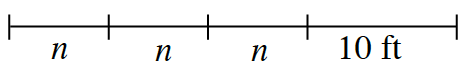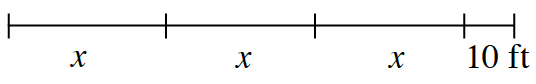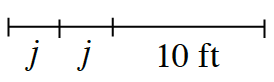### Home > CC1 > Chapter Ch5 > Lesson 5.1.2 > Problem5-16

5-16.

Kelani wants to cut a piece of rope into several equally-sized pieces and then have a 10-foot piece remaining. Write an algebraic expression to represent the length of each rope shown in the diagrams below. Then use the equation you create to help Kelani figure out how long to make each of the equally-sized pieces. Homework Help ✎

1. A 25-foot piece of rope (find n).• The equation 3n + 10 = 25 represents this length of rope.
Now solve the equation to find n.

n = 5 feet

• Subtract 10 from both sides. 3n = 15

• Divide each side by 3.

• $\frac{3n}{3}=\frac{15}{3}$

1. A 310-foot piece of rope (find x).• You can use the equation 3x + 10 = 310 to represent this length of rope.
Follow the steps outlined in part (a) to solve for x.

1. A 13-foot piece of rope (find j).• Can you write your own equation for this rope?
Remember that there are only 2 j's and the total length is 13 feet.